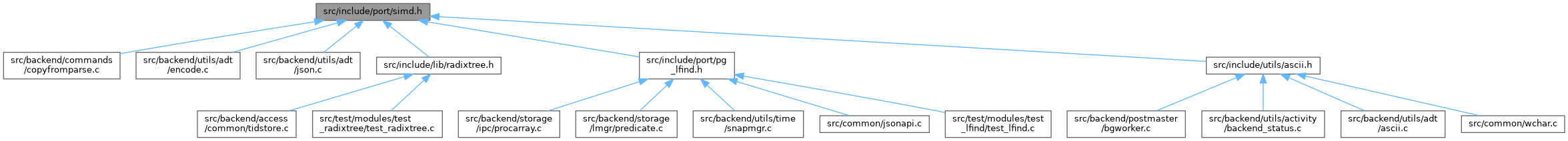PostgreSQL Source Code  git master
simd.h File Reference
This graph shows which files directly or indirectly include this file:Go to the source code of this file.

## Macros

#define USE_NO_SIMD

## Typedefs

typedef uint64 Vector8

## Functions

static void vector8_load (Vector8 *v, const uint8 *s)

static Vector8 vector8_broadcast (const uint8 c)

static bool vector8_has (const Vector8 v, const uint8 c)

static bool vector8_has_zero (const Vector8 v)

static bool vector8_has_le (const Vector8 v, const uint8 c)

static bool vector8_is_highbit_set (const Vector8 v)

static Vector8 vector8_or (const Vector8 v1, const Vector8 v2)

## ◆ USE_NO_SIMD

 #define USE_NO_SIMD

Definition at line 59 of file simd.h.

## ◆ Vector8

 typedef uint64 Vector8

Definition at line 60 of file simd.h.

## Function Documentation

 static Vector8 vector8_broadcast ( const uint8 c )
inlinestatic

Definition at line 133 of file simd.h.

134 {
135 #if defined(USE_SSE2)
136  return _mm_set1_epi8(c);
137 #elif defined(USE_NEON)
138  return vdupq_n_u8(c);
139 #else
140  return ~UINT64CONST(0) / 0xFF * c;
141 #endif
142 }
char * c

Referenced by vector8_has(), vector8_has_le(), and vector8_is_highbit_set().

## ◆ vector8_has()

 static bool vector8_has ( const Vector8 v, const uint8 c )
inlinestatic

Definition at line 160 of file simd.h.

161 {
162  bool result;
163
164  /* pre-compute the result for assert checking */
165 #ifdef USE_ASSERT_CHECKING
166  bool assert_result = false;
167
168  for (Size i = 0; i < sizeof(Vector8); i++)
169  {
170  if (((const uint8 *) &v)[i] == c)
171  {
172  assert_result = true;
173  break;
174  }
175  }
176 #endif /* USE_ASSERT_CHECKING */
177
178 #if defined(USE_NO_SIMD)
179  /* any bytes in v equal to c will evaluate to zero via XOR */
180  result = vector8_has_zero(v ^ vector8_broadcast(c));
181 #else
183 #endif
184
185  Assert(assert_result == result);
186  return result;
187 }
unsigned char uint8
Definition: c.h:493
size_t Size
Definition: c.h:594
int i
Definition: isn.c:73
Assert(fmt[strlen(fmt) - 1] !='\n')
Definition: simd.h:133
static bool vector8_has_zero(const Vector8 v)
Definition: simd.h:193
uint64 Vector8
Definition: simd.h:60
static bool vector8_is_highbit_set(const Vector8 v)
Definition: simd.h:269

References Assert(), i, vector8_broadcast(), vector8_has_zero(), and vector8_is_highbit_set().

Referenced by pg_lfind8(), and vector8_has_zero().

## ◆ vector8_has_le()

 static bool vector8_has_le ( const Vector8 v, const uint8 c )
inlinestatic

Definition at line 211 of file simd.h.

212 {
213  bool result = false;
214
215  /* pre-compute the result for assert checking */
216 #ifdef USE_ASSERT_CHECKING
217  bool assert_result = false;
218
219  for (Size i = 0; i < sizeof(Vector8); i++)
220  {
221  if (((const uint8 *) &v)[i] <= c)
222  {
223  assert_result = true;
224  break;
225  }
226  }
227 #endif /* USE_ASSERT_CHECKING */
228
229 #if defined(USE_NO_SIMD)
230
231  /*
232  * To find bytes <= c, we can use bitwise operations to find bytes < c+1,
233  * but it only works if c+1 <= 128 and if the highest bit in v is not set.
235  * https://graphics.stanford.edu/~seander/bithacks.html#HasLessInWord
236  */
237  if ((int64) v >= 0 && c < 0x80)
238  result = (v - vector8_broadcast(c + 1)) & ~v & vector8_broadcast(0x80);
239  else
240  {
241  /* one byte at a time */
242  for (Size i = 0; i < sizeof(Vector8); i++)
243  {
244  if (((const uint8 *) &v)[i] <= c)
245  {
246  result = true;
247  break;
248  }
249  }
250  }
251 #else
252
253  /*
254  * Use saturating subtraction to find bytes <= c, which will present as
255  * NUL bytes. This approach is a workaround for the lack of unsigned
256  * comparison instructions on some architectures.
257  */
259 #endif
260
261  Assert(assert_result == result);
262  return result;
263 }

References Assert(), i, vector8_broadcast(), and vector8_has_zero().

Referenced by pg_lfind8_le(), and vector8_has_zero().

## ◆ vector8_has_zero()

 static bool vector8_has_zero ( const Vector8 v )
inlinestatic

Definition at line 193 of file simd.h.

194 {
195 #if defined(USE_NO_SIMD)
196  /*
197  * We cannot call vector8_has() here, because that would lead to a
198  * circular definition.
199  */
200  return vector8_has_le(v, 0);
201 #else
202  return vector8_has(v, 0);
203 #endif
204 }
static bool vector8_has_le(const Vector8 v, const uint8 c)
Definition: simd.h:211
static bool vector8_has(const Vector8 v, const uint8 c)
Definition: simd.h:160

References vector8_has(), and vector8_has_le().

Referenced by vector8_has(), and vector8_has_le().

## ◆ vector8_is_highbit_set()

 static bool vector8_is_highbit_set ( const Vector8 v )
inlinestatic

Definition at line 269 of file simd.h.

270 {
271 #ifdef USE_SSE2
273 #elif defined(USE_NEON)
274  return vmaxvq_u8(v) > 0x7F;
275 #else
277 #endif
278 }

Referenced by vector8_has().

 static void vector8_load ( Vector8 * v, const uint8 * s )
inlinestatic

Definition at line 106 of file simd.h.

107 {
108 #if defined(USE_SSE2)
109  *v = _mm_loadu_si128((const __m128i *) s);
110 #elif defined(USE_NEON)
111  *v = vld1q_u8(s);
112 #else
113  memcpy(v, s, sizeof(Vector8));
114 #endif
115 }

Referenced by pg_lfind8(), and pg_lfind8_le().

## ◆ vector8_or()

 static Vector8 vector8_or ( const Vector8 v1, const Vector8 v2 )
inlinestatic

Definition at line 306 of file simd.h.

307 {
308 #ifdef USE_SSE2
309  return _mm_or_si128(v1, v2);
310 #elif defined(USE_NEON)
311  return vorrq_u8(v1, v2);
312 #else
313  return v1 | v2;
314 #endif
315 }